metamerist

Wednesday, September 27, 2006

Linear Algebra for Graphics Geeks (Rotation)

Todd Will's excellent Introduction to the Singular Value Decomposition contains a some good insights regarding rotation matrices. Those insights are the inspiration behind this post.

Consider the 2x2 rotation matrix: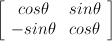What's the result if we multiply by the identity matrix?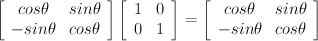It's a silly question for anyone with a basic understanding of linear algebra. Multiplying by the identity matrix is the linear algebra equivalent of multiplying by one. Any matrix multiplied by the identity matrix is itself, A*I=A.

That said, let's look at things in a new light. Consider the rotation matrix transforming two column vectors, a unit vector on the x axis (1,0) and a unit vector on the y (0,1) axis:The funny thing is that this is the same problem--the matrix containing the two column vectors (1,0) and (0,1) is equal to the identity matrix.

From the perspective of transforming these two column vectors, it's clear that the transformation rotates (1,0) into alignment with the first column vector and (0,1) into alignment with the second column vector.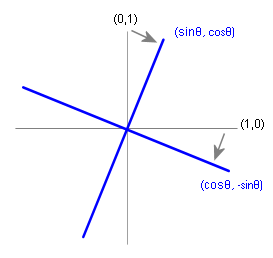Because the matrix is orthogonal, its inverse is its transpose. Multiplying the 2D rotation matrix by its transpose gives us: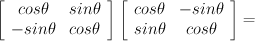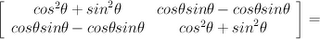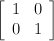The moral of the story is the effect of a matrix with an orthonormal basis in its column space. When the column vectors are orthogonal, the effect of the transformation is well understood by considering the transformation of the identity matrix, which is itself another orthonormal basis. The vector space represented by the identity matrix is rotated into alignment with the vector space defined by the orthonormal basis in the column vectors of the rotation matrix.

Another point for consideration is the effect of transposing the rotation matrix; the transpose of the rotation matrix is its inverse, and transposing it has the effect of turning the column vectors into row vectors; when the orthonormal basis of the column space is transposed into the row space, the effect is opposite that of the original matrix: the vector space defined by orthonormal basis in the row vectors is brought into alignment with the standard axes defined by the identity matrix.

Read more on Todd Will's page titled Perpframes, Aligners and Hangers. It's part of his tutorial on SVD, and it's a nice aid in comprehension.

This is post #11 of Linear Algebra for Graphics Geeks. Here are links to posts #1, #2, #3, #4, #5, #6, #7, #8, #9, #10.

Next, some notes on hand calculation of the SVD.

Tuesday, September 26, 2006

In the Clouds

When it comes to Scilab (or Matlab), I'm far from being an expert. That probably shouldn't be the case, but this is an area where I have so much of my own code accumulated that I've always wound up either rolling my own solution or plunking away with spreadsheet matrix functions. But I'm learning, and if you'd like to learn Scilab/Matlab too, I'm slowly accumulating simple examples here.

This evening I cranked out a few simple Scilab functions to generate random point clouds that will hopefully provide support for a future post on PCA. They're available on this page along with some very simple functions to plot points and rotate and scale in 2D. If you have no interest in the results generated, they still may be helpful in showing how to create simple functions in Scilab.

Viral Causes

Here in Minnesota there are instances where you can be fully reimbursed for donations made to political candidates. While mulling the prospects of sending friendspam encouraging support for candidates and reminding friends a contribution would cost them nothing, the thought of marrying social causes and social software crossed my mind. Maybe it's already been done, but I haven't seen anything like what I have in mind. Here are a few thoughts on the subject.

One objective of such a system would be to increase involvement in causes by making the effect of individual action evident. It's very disconcerting when you feel you're not making a difference, but it's very energizing when you do feel you're making a difference. The right visualization could show people they are making a difference and encourage them to make an even bigger difference. I'd like to see a visualization showing my effect on first degree contacts, second degree contacts, etc. Show me I made a difference!

In the case of fundraising, totals collected could be shown. Contests could be managed as well. It might work similar to Evite, except the focus would be around causes and fundraising rather than events. A truly successful site might wind up becoming the portal of choice for charitable contributions.

\$0.02

Clay and Form

A couple days ago, I mentioned my being labelled a "syntactic sugar" guy due to my alleged lack of in sufficient zeal for new languages. (Strongtalk looks interesting to me.)

As I see it, languages are the clays of computing. Some clays are more suitable than others in the creation of various forms, some clays have interesting properties, but I tend to find the form more interesting than the clay.

On this note, Bernard Chazelle's updated essay The Algorithm: Idiom of Science.

(via Geomblog)

Monday, September 25, 2006

Couple on Bench in Riomaggiore

The road to wisdom? -- Well, it's plain
and simple to express:
Err
and err
and err again
but less
and less
and less.

-- Grooks, by Piet Hein

Sunday, September 24, 2006

Reflecting on Languages

Refections on experiences with programming languages...

It's a familiar story. A kid in the 80s stumbles upon a TRS-80, an Apple II or an Atari 800, opens to the "Hello World" page of the manual, and the rest is history. It's my story too. Programming, the way it began.

As far as languages go, unsurprisingly, BASIC was first. I was another one of those kids tapping away on an Atari computer. My brother and I had a simple game up and running on the first day. I could reflect on experiences with my first computer to great length, but this post is about programming languages, so I'll carry on.

Subsequent experiences with programming languages ranged from dabblings to wholesale conversions. Some experiences were the result of assigned classwork. Other experiences came in response to needs and curiosities.

I had access to a timesharing system in high school, which offered me the chance to experiment with several languages the system supported: BASIC, Pascal, Snobol, Fortran, etc.

Fortran? It was worthy of experimentation, but not without its irritations (like syntax determined by whitespace, a phenomenon for which there's absolutely no excuse nowadays!).

As far as languages beginning with the letters F-O-R go, there was also Forth. I purchased a copy for my Atari and spent quite a bit of time playing with it. I didn't know it at the time, but it helped prepare me for later work with PostScript.

A desire to create better video games led me into the world of 6502 assembly language. It was the only way to get the speed. There's something immensely satisfying about working in assembly language, especially to those who enjoy puzzles and challenges--breaking higher concepts down into primitive bit-twiddling instructions, getting right down to the metal, honing the inner loops, watching each step of the cpu's gears.

Experience with Pascal eventually translated into a wholesale conversion--it became my language of choice, largely due to Turbo Pascal, Borland's fast and fine compiler at the time. In terms of structure and performance it was an enormous improvement over BASIC.

After playing around with a half a dozen languages in high school, college offered me fewer surprises than I expected. I developed a great admiration for Lisp. Several of my courses used it, and I did quite a bit of recreational programming in it as well. Of all the languages I learned in college, it was the most mind expanding. My appreciation for it generated many shrugs over the years, and I offer thanks to Paul Graham for making it cool to be a Lisp aficionado. :)

Cobol? Cursed Cobol. It was a class so mind-numbing and tedious I wrote a code generator for it. Rather than plunking in the Cobol to generate fictitious corporate reports, I plunked in a sample report and let the code generator spew out the pages of carefully spaced Cobol needed to generate it. It saved me hours and hours of work in a single class. Ugh!

Aside from Lisp, another college language that left a significant impact on me was Ada. I still retain a good deal of respect for the language. It had nice support for object-oriented programming, generic programming, type safety, multi-threading. For a long time, it was a point of reference in the critique of languages arriving afterwards.

Other languages learned in college include x86 assembly language, something I still use when needed. Prolog was interesting, and it offered interesting insights, but I never found any use for it professionally. I also enjoyed playing with a language called Rexx. Unfortunately, I never ran into Smalltalk in college, and as far as omissions go, this one is most unfortunate.

C eventually replaced Pascal as my language of choice. After that, it was C++ as soon as Borland's Turbo C++ became available.

And then there's Java, Javascript, Python, PHP and other languages resulting from the rise of the Internet.

Insights and conclusions? This is something I'm going to be thinking about in greater depth.

A longtime friend and ex-colleague of mine is still a language fiend by my estimation. From his perspective, I'm a "syntactic sugar" guy, meaning I see many hailed improvements in programming languages as mere refinements in syntax rather than more desirable substantial improvements.

As far as metaphors go, I prefer "rearranging the syntactic furniture":

"Oh, let's put that over here now!"
"Why?"
"It will be fun!"

What's most frustrating is that many alleged improvements in new languages appear to be steps backward; especially syntax determined by white space, which strikes me as the geek equivalent of bringing back bad fashion ideas from the 70s and 80s. Pet rock, anyone?

Saturday, September 23, 2006

LassieA Metacritic 84 prompted me to take the gang out to see Lassie, and all I can say is bravo to Peter O'Toole, Samantha Morton, Peter Dinklage, Jonathan Mason, Hester Odgers, director Charles Sturridge and the rest of the cast for a truly, wonderful film; casting, acting, writing, cinematography, direction... everything was well done in what is the most satisfying family film I've seen since Whale Rider.

"'Lassie' balances cruelty and tenderness, pathos and humor without ever losing sight of its youngest audience member." -- Jeanette Catoulis, NYT

Friday, September 22, 2006

Organic BalanceSo many posts about mathematics demand some sort of cosmic balance--like this sunflower shot I took earlier this week.

Linear Algebra for Graphics Geeks (SVD-VIII)

The SVD and the Moore-Penrose Pseudoinverse

Let's look at the SVD and its inverse.

A = USVT

A-1 = (USVT)- 1 =
(VT)-1S-1U-1 =
VS-1UT

Here's the question for this post:

If we substitute the pseudoinverse of S for S-1 in the product above, will we wind up with the pseudoinverse of A?

In other words, is A+ = VS+UT?

I've read quite a bit on the subject in the past few months, but I don't recall seeing this worked out, so I'm going to do it just for the sake of sanity and entertainment. :)

A few reminders for the sake of clarity:

First, because U and V are orthogonal matrices their inverses and tranposes are the same, which is why either matrix cancels out its transpose (UUT=UTU=VVT=VTV=I). Second, there are several simplifications involving S and S+ that exploit the identities of pseudoinverses. I'll note these when they are applied. Also, the inverse of a matrix product is the reverse product of the individual inverses (likewise with transposes). cf. The Matrix Cookbook.

Matrix A needs to meet four criteria to be a pseudoinverse. Let's substitute USVT for A and VS+UT for A+ and see if everything works out.

Rule #1:

AA+A = A

(USVT)(VS+UT) (USVT) =
US(VTV)S+(UTU) SVT =
U(SS+S)VT =
USVT

note: The last step applies this rule (#1) to (SS+S), which we can do because S+ is a pseudoinverse.

Rule #2:

A+AA+ = A+
(VS+UT)(USVT)(VS+UT) =
VS+(UTU)S(VTV) S+UT =
V(S+SS+)UT =
VS+UT

note: The last step applies this rule (#2) to (S+SS+), which we can do because S+ is a pseudoinverse.

Rule #3:

(AA+)T = AA+

AA+ = (USVT)(VS+UT)
AA+ = USVTVS+UT
AA+ = US(VTV)S+UT
AA+ = USS+UT
AA+ = U(SS+)UT

(AA+)T = (U(SS+)UT)T
(AA+)T = UTT(SS+)TUT
(AA+)T = U(SS+)TUT
(AA+)T = U(SS+)UT

note: The last step applies this rule (#3) to (SS+)T, which we can do because S+ is a pseudoinverse.

Rule #4:

(A+A)T = A+A

A+A = (VS+UT)(USVT)
A+A = VS+(UTU)SVT
A+A = V(S+S)VT

(A+A)T = (V(S+S)VT)T
(A+A)T = VTT(S+S)TVT
(A+A)T = V(S+S)TVT
(A+A)T = V(S+S)VT

note: The last step applies this rule (#4) to (S+S)T, which we can do because S+ is a pseudoinverse.

Finally, let's look at an identity mentioned in a my last post on the subject. This is an important one relating to least squares.

A+ = (ATA)-1AT
A+ = ((USVT)T(USVT))-1(USVT)T
A+ = ((VSTUT)(USVT))-1(VSTUT)
A+ = (USVT)-1(VSUT)-1(VSUT)
A+ = (USVT)-1
A+ = (VT)-1S+U-1
A+ = VS+UT

fin

This is post #10 of Linear Algebra for Graphics Geeks. Here are links to posts #1, #2, #3, #4, #5, #6, #7, #8, #9.

Next: Some insights relating to rotation matrices.

Wednesday, September 20, 2006

PeakStream unveils multicore and CPU/GPU programming solution

Stanford's Pat Hanrahan (Brook GPU) is now chief scientist at a GPGPU-related start up with \$17M in funding and some experienced techies.

Ars Technica:

"Today marks the official launch of PeakStream, a software start-up that has been operating in stealth mode for over a year now while developing a new type of software platform aimed at making multiprocessor systems easier to program. PeakStream's product, which I'll describe in more detail in a moment, is basically a set of tools—APIs, a virtual machine, a system profiler, a JIT compiler, etc.—that present a standardized, stream-processing-based programming model for which programmers can develop multithreaded applications. A program written to PeakStream's APIs can be compiled once and run on a variety of multiprocessing platforms, including multicore x86 processors, CPU + GPU combinations, and eventually even IBM's Cell. The PeakStream Platform's VM handles all of the scheduling and resource allocation behind the scenes, fitting the application to each system's particular mix of hardware at runtime."

Tuesday, September 19, 2006

Linear Algebra for Graphics Geeks (Pseudoinverse)

This is post #9 of Linear Algebra for Graphics Geeks. Here are links to posts #1, #2, #3, #4, #5, #6, #7, #8.

This post is on the Moore-Penrose pseudoinverse of a matrix. I'm going to present this in ways I've never seen it presented, so wish me luck.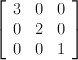It scales x by 3, y by 2, and it leaves z as it is.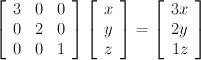This is a scale matrix, a diagonal matrix. It can be used to transform from one 3D space to another 3D space that is three times as wide and twice as high. It's a nonsingular matrix. There's not reduction in dimensionality. It's invertible.

The inverse of a diagonal matrix is trivial. Each element on the diagonal is replaced by its reciprocal. Here's the inverse of our matrix.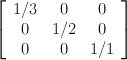And, here it is in action.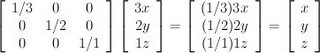In the last post on linear algebra, I discussed "the column view" and presented some examples in which columns of the identity matrix were zeroed out. Let's do something similar and zero out the last column of our matrix.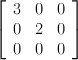The effect of this matrix is much the same as the original matrix, x is tripled and y is doubled, but this time z is zeroed; that is, everything is scaled in x and y and then projected onto the x-y plane.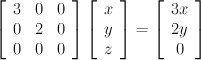This matrix is singular. It's not invertible. If you read the last post on this subject, it should be clear the dimensionality of the column space is 2 and that this matrix is less than full rank.

If we try to invert this diagonal matrix, we'll divide by zero when we try to replace the element in the third column with its reciprocal, but here's the big question:

What if we invert the elements we can invert (the non-zero elements) and forget about the elements we can't invert (the zeroes)?

If we do that, we'll wind up with this matrix: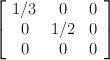Let's try using this "we-inverted-what-we-could matrix" as a substitute inverse.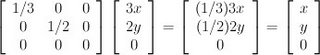Conclusion? We got x back to its original state. We got y back too. On the other hand, z's still zero, but what can we expect? Once you've multiplied by zero, there's no way to go back. Everything projected onto the x-y plane in the original transformation stays there.

Let's look at everything from another perspective. In the previous post, I showed how the product of a matrix and a vector results in a linear combination of the column vectors of the matrix--in other words, the product is always in the column space of the matrix.

In the case of the matrix that's the subject of this post, we calculated the inverse as best we code, inverting the elements of a diagonal matrix where possible. When we used this substitute inverse, we saw some of the components restored; the ones restored were in the column space of the original matrix--the z values weren't in the original column space, and they weren't restored.

The substitute inverse we calculated is an example of a Moore-Penrose pseudoinverse, and it's often just referred to as a pseudoinverse. It's denoted with a superscripted plus sign, as in the following.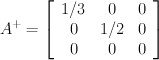A Moore-Penrose pseudoinverse must meet the following criteria:

1. AA+A = A
2. A+AA+ = A+
3. (AA+)T = AA+
4. (A+A)T = A+A

(The T indicates tranposes. I have been using apostrophes, but I'm concluding they're hard to see.)

There's a lot to be said about pseudoinverses (see Wikipedia, Mathworld). Here are a few key points.

1. Pseudoinverses may be rectangular (unlike ordinary inverses which must be square).
2. When a matrix is square and invertible, its inverse and pseudoinverse are equal.
3. If (ATA) is invertible, then A+ = (ATA)-1AT. (Important fact for least squares fit!)
4. Given, Ax = b, the pseudoinverse provides the least squares solution with x = A+b.
5. The SVD is the most common means of calculating pseudoinverses (next post).

Next: The SVD and the Moore-Penrose Inverse.

Imaginary WorldWandered through Mill Ruins Park the other night. Lately, this city has been noteworthy as far as architecture goes. (Yey for us!) The photo above is the new Guthrie theater reflected on the murky, warpy waters of the Mississippi.

Monday, September 18, 2006

Linear Algebra For Graphics Geeks (The Column View)

Finding myself in the mood for a brief return to linear algebra.

This is post #8 of Linear Algebra for Graphics Geeks. Here are links to posts #1, #2, #3, #4, #5, #6, #7.

The point of this post is something called "the column view" of matrices and matrix products. Very frequently, linear algebra offers multiple perspectives of the same mathematical phenomenon; each perspective offers new insight; "the column view" is such a perspective.

Consider the transformation of the vector [x y z] by the following matrix.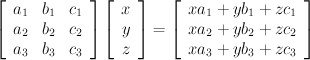The "column view" offers the insight that the product is a linear combination of the columns of the matrix, each column is weighted by x, y and z, respectively.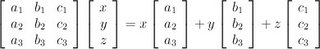If the N columns of a square matrix form a basis for RN, then the dimension of the column space is N. At the other extreme, if every element of the matrix is zero, the only possible result of any product is a zero-dimensional result, all zeroes.

In the 3D case, if the matrix is all zeroes, the product is [0 0 0] regardless of the value of [x y z]. Given a matrix with N columns, the possible dimensionality of transformed vectors can be anywhere in the range from 0 to N.

This is one of those aspects of linear algebra that can be a duh! or a revelation depending on one's experiences.

From the perspective of the "column view," the identity matrix is an orthonormal basis for Rn. In the case of R3, below, the first column is a unit vector along the x-axis, the second column is a unit vector along the y-axis and finally the last column is a unit vector along the z-axis. The effect of the identity matrix on vectors, of course, is no change.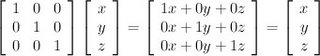From the "column view" perspective, it's quite clear you can go anywhere in R3 via combinations of the columns of the identity matrix--every transformation is a combination of the columns of the identity matrix and any point in R3 going into the transformation is the same coming out of the transformation.

If we completely zero out one of the columns in the identity matrix by replacing a one with a zero, the effect is quite clear: we'll lose a dimension. For example, in the following, you can see that every [x y z] will be projected onto the x-y plane regardless of the value of z. In this case, the column space is 2D.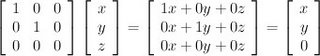Likewise, if we zero yet another column, we'll reduce the dimensionality of the column space down to 1D, with the effect being every vector being reduced to its x component.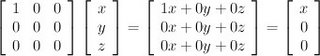Finally, we've already discussed the case where a matrix is all zeroes.

A key point here is that when we transform a vector with a matrix, the resulting vector is always in the column space of the matrix; the dimensionality of the column space determines the dimensionality of the results.

Next: The Pseudoinverse.

Sunday, September 17, 2006

When All's Well

Still enjoying the video treasure trove that is YouTube.This link, an old video for When All's Well from Everything But the Girl's 1985 release Love Not Money. Although the disc is a top favorite in my collection, it was pretty much unknown here in the U.S. outside music geek circles. This is early EBTG, 10 years prior to their hit Missing.

Love Not Money was released at a time when there were strong country and rockabilly influences on the British music scene, which made for an interesting combination with lead singer Tracey Thorn's punked out hair and the beautiful harmonics in her lush voice.

Tracks exhibiting the heaviest country influences include Anytown, Ugly Little Dreams and Are You Trying to Be Funny? In addition, tracks such as Shoot Me Down and This Love (Not For Sale) bring the wonderful contrast that makes it a personal favorite.

Thursday, September 07, 2006

Taking a Break

The recent posts I've made regarding linear algebra have generated an unexpected amount of traffic. While I hadn't planned on writing as much as I did on the subject of the Singular Value Decomposition, what frustrates me is that I feel there's so much more that needs to be said. Especially the following:

1. The relationship between the SVD and the Moore-Penrose Pseudoinverse.
2. The relationship between SVD and PCA and dimensionality reduction.
3. The relationship between SVD and general least squares.

For now, I need to attend to other things and take a break. If you've been at all intrigued by the series so far, you may want to do more research on the three cases listed above.

Tuesday, September 05, 2006

Linear Algebra for Graphics Geeks (SVD-VII)

This is post #7 of Linear Algebra for Graphics Geeks. Here are links to posts #1, #2, #3, #4, #5, #6. The subject at hand is the Singular Value Decomposition.

Today, I'm going to discuss the SVD of the following matrix.

1 2 3 4 5
1 2 3 4 5
1 2 3 4 5
1 2 3 4 5
1 2 3 4 5

Looking at this matrix a couple of things should be obvious. First, because rows are duplicated, it's singular. Also, since every row of the matrix is duplicated, the rank is very low.

Let's look at the SVD of this matrix ([1 1 1 1 1]'*[1 2 3 4 5] results in the matrix above).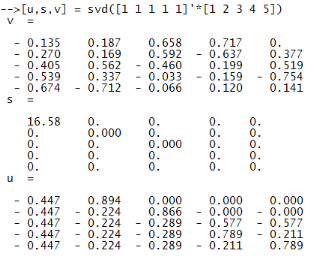Because there's only one non-zero element on the diagonal of S, we see that our matrix is indeed singular and that it has a rank of 1.

The columns of U and V are associated with the singular values on the diagonal of S. Zero elements on the diagonal of S do not contribute to the result (since they're multiplied by zero); consequently, the corresponding columns of U and V can be discarded.

In addition to A=U*S*V', we've looked at the SVD in the form A*V=U*S. Another valid way of looking at the equation is the following:

A*Vi = Sii * Ui

Where Vi is the i-th column of V, Sii is an element on the diagonal of S and Ui is the i-th column of U. From this perspective, A transforms the column vectors of V to produce the column vectors of U scaled by S. Viewed in this light, I think it's clearer that columns can be discarded when the corresponding elements in S are zero.

In the SVD calculated above, there was only one non-zero element on the diagonal of S. Let's discard columns associated with zeroes and look at the product of the first column of U, the first (top-left) element of S and the first column of V.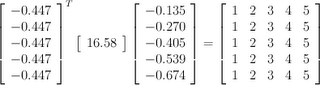The results, of course, aren't quite perfect. In reality, there's some minute loss due to error, but it's minimal, so I've reproduced the original matrix exactly for the sake of clarity.

If you imagine the elements of our matrix to be pixels, you're well on your way to understanding how the SVD can be used for image compression. Given the SVD of a block of pixels, one can set a threshold on the elements of S and keep only the most significant elements of S and the associated columns of U and V, in much the same way that the significant Discrete Cosine Transform coefficients are retained in JPEG compression.

If you search the Internet for the terms "SVD" and "image compression," you'll find a great deal of information on the subject. One excellent SVD tutorial is Todd Will's Introduction to the Singular Value Decomposition. It contains an example of using SVD for compression. Also, on a similar note, the said properties of the SVD are useful in extracting features for face recognition (for more info, search for "eigenfaces and SVD").

One more thing. If you've been reading these posts on the SVD, and you'd like more information, another excellent paper on the subject is Dan Kalman's A Singularly Valuable Decomposition: The SVD of a Matrix.

My next linear algebra post is The Column Space.

Friday, September 01, 2006

Linear Algebra for Graphics Geeks (SVD-VI)

This is post #6 of Linear Algebra for Graphics Geeks. Here are links to posts #1, #2, #3, #4, #5. The subject at hand is the Singular Value Decomposition.

This time I'm going to discuss eigenvectors, eigenvalues and ways in which they are related to the SVD.

For all the complaints levied against Wikipedia, I am often pleased by how clearly and succinctly many subjects are explained:

"An eigenvector of a transformation is a non-null vector whose direction is unchanged by that transformation. The factor by which the magnitude is scaled is called the eigenvalue of that vector"

Let's say you have a matrix A and a vector V and you transform V with A. If A has no effect on the direction of V and all that A does is make V longer or shorter (or the same), then V is an eigenvector of A:

A*V = scale*V

The value of "scale" is the eigenvalue for the eigenvector V. Eigenvalues are usually identified with the symbol lamba.

Take our matrix that scales X by 3 and Y by 2:

[3 0]
[0 2]

The eigenvectors of this matrix are [1 0]' and [0 1]'. The eigenvalues are 3 and 2.

[3 0] *  =  = 3 * 
[0 2] .  .  . . . 

[3 0] *  =  = 2 * 
[0 2] .  .  . . . 

Transformation of the unit vector on the x axis [1 0]' results in [3 0]', a 3X scaling of its length. Likewise, transformation of the unit vector on the y axis [0 1]' results in [0 2]', a 2X scaling of its length.

The eigen decomposition takes the following form:

A = P*D*P-1

Where P is an orthogonal matrix and D is a diagonal matrix.

This decomposition can always be done on a symmetric matrix (Spectral Theorem). Since the product of a matrix and its transpose is always a symmetric matrix, this decomposition can always be done on A*A' and A'*A.

Note to self: In a revision, I'd like to say something about the relationship between the eigen decomposition and the ellipse equation. One form of the equation for the ellipse is ax2 + bx2 = 1.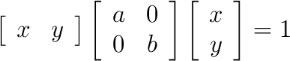While we're on the subject, it's interesting to consider the the square of a symmetric matrix given its eigen decomposition.

A2 = A*A = (P*D*P-1)*(P*D*P-1)
= P*D*P-1*P*D*P-1
= P*D*(P-1*P)*D*P-1
= P*D*I*D*P-1
= P*D*D*P-1
= P*D2*P-1

Note that squaring the matrix results in a squaring of D, and since D is a diagonal matrix, this amounts to a squaring of each element on D's diagonal.

We've gotten far enough to note more interesting properties of SVD:

1. The columns of U are the eigenvectors of A*A'
2. The columns of V are the eigenvectors of A'*A
3. The diagonal elements of S are the square roots of the eigenvalues of A*A' and A'*A.

Like many other proporties of the SVD laid out so far, these three facts also have profound implications. Hopefully, I'll have time to look into it much more deeply in a future post, but I think everything above is more than enough to ponder for now.

I'll leave with some equations that get us from the SVD to the eigen decompositions of A*A' and A'*A.

A = U*S*V'

A' = (U*S*V')'
A' = V*S*U'

A*A' = (U*S*V')*(V*S*U')
= U*S*V'*V*S*U'
= U*S*(V'*V)*S*U'
= U*S*I*S*U'
= U*S2*U'

A'*A = (V*S*U')*(U*S*V') =
V*S*U'*U*S*V' =
V*S*(U'*U)*S*V' =
V*S*I*S*V' =
V*S2* V'

Note: This is true when A is rectangular as well as square.

In part #7, I look at the SVD of a singular matrix.